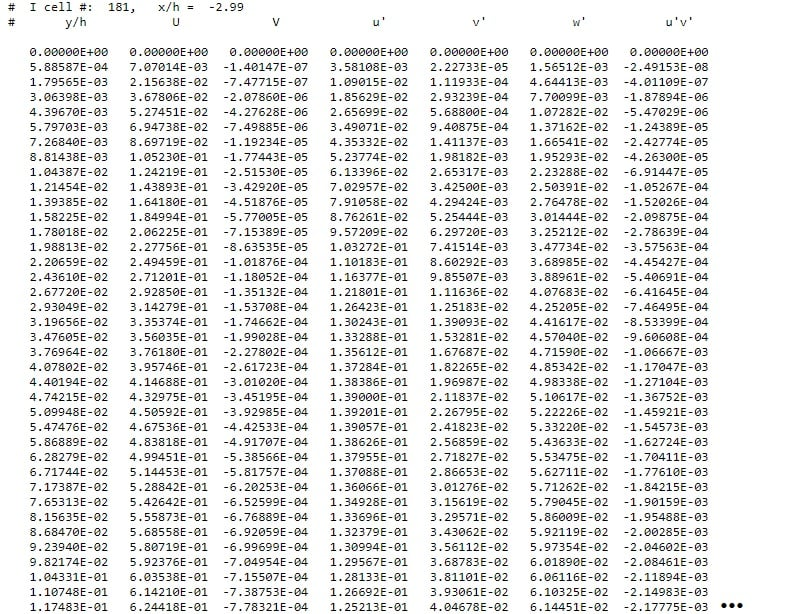José Mantovani
Subscriber

Hello Br Bell!

Just today I had time to see this, I make other projects.

So Can you give me some help?

I caugth the profile txt file from a ANSYS FLUENT solution to change the parameters as we discussed through this thread. So here I have a file with y, U, V, k and eps from FLUENT. From what we discussed I think I need replace the values of U and V correlating with the y values. In image below have the last data that I said to you and you checked Br Bell. I need multiply the y/h column per h value to return to y dimension and the values u', v', w' multiply by Uo do return to true values, as you said. But, my doubt is: in txt profile that I got in FLUENT have the values of k and eps and not to u', v', w'. Below have image of values that I said.According to the literature the formula to k is k= 1/2 (u'^2+v'^2+w'^2) so I need correlate for first y value the values of first line of "column 1" (already multiplied by Uo) and add them (sum) and multiply by 1/2, its correct? And another doubt is about epsilon, How is the formula of epsilon? If I use the formula that you posted I need the values of Cmhu and vt and I think this way is more complicated, have other way to get the epsilon values? Or, how to get a file from FLUENT with the values separated? There's a possibility? Maybe a txt file from a LES solution?

Thanks for helping. I'm waiting here.

Mantovani.